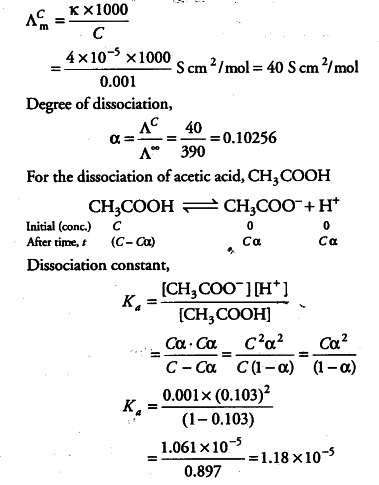# Calculate the dissociation constant of acetic acid

Calculate the dissociation constant of acetic acid, if molar conductivity at infinite dilution for acetic acid is 390 S \${{cm}^{2}}\$/mol.

Molar conductivity at 0,001 M,